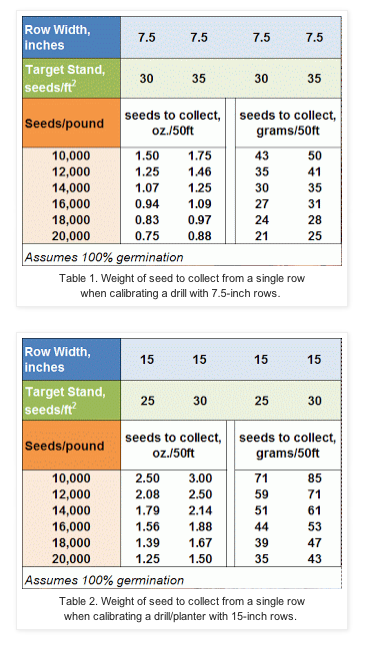Source: Chad Lee, Extension Agronomist, University of Kentucky

As producers gear up for wheat planting, calibration of the seeder is extremely important. Below are two tables to use as quick references in the calibration process. Most producers are using drills with 7.5-inch row spacing. The first table calculates how much seed to collect in 50 linear feet from one row of the drill. Target populations of 30 and 35 seeds per square foot are used for the calculations. The needed seed weights are listed as ounces (oz.) and as grams.

The second table calculates how much seed to collect in 50 linear feet of one row from a drill (or planter) with 15-inch rows. Target populations of 25 and 30 seeds per square foot are used. The needed seed weights are listed in both ounces and grams.In both of these tables, 100% germination is assumed. Most seed is not at 100% germination and the final seed weight to collect can be adjusted by the standard germination value. The adjustment occurs by dividing the seed weight by the percentage of the standard germination.

For example, if producer has a seed lot of 10,000 seeds per pound and she wants to plant in 7.5-inch rows, with a target population of 35 seeds per square foot, then she needs to collect 1.75 oz (50 grams) per 50 foot of each row. But, if the standard germination is 95%, then she needs to divide 50 grams by 0.95 which equals 52.6 grams (50 / 0.95 = 52.6).

For more details about how to calculate wheat drills, go to: http://www2.ca.uky.edu/agc/pubs/id/id125/04.pdf